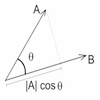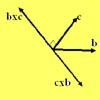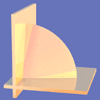Search by Topic

Resources tagged with Vector products similar to Cross with the Scalar Product:

Filter by: Content type:
Age range:
Challenge level:

There are 5 results

Broad Topics > Vectors and Matrices > Vector productsCross with the Scalar Product

Age 16 to 18 Challenge Level:

Explore the meaning of the scalar and vector cross products and see how the two are related.Multiplication of Vectors

Age 16 to 18

An account of multiplication of vectors, both scalar products and vector products.V-P Cycles

Age 16 to 18 Challenge Level:

Form a sequence of vectors by multiplying each vector (using vector products) by a constant vector to get the next one in the seuence(like a GP). What happens?Quaternions and Reflections

Age 16 to 18 Challenge Level:

See how 4 dimensional quaternions involve vectors in 3-space and how the quaternion function F(v) = nvn gives a simple algebraic method of working with reflections in planes in 3-space.Walls

Age 16 to 18 Challenge Level:

Plane 1 contains points A, B and C and plane 2 contains points A and B. Find all the points on plane 2 such that the two planes are perpendicular.